# AP SSC 10th Class Maths Solutions Chapter 14 Statistics Ex 14.3

AP State Board Syllabus AP SSC 10th Class Maths Textbook Solutions Chapter 14 Statistics Ex 14.3 Textbook Questions and Answers.

## AP State Syllabus SSC 10th Class Maths Solutions 14th Lesson Statistics Exercise 14.3

### 10th Class Maths 14th Lesson Statistics Ex 14.3 Textbook Questions and Answers

Question 1.
The following frequency distribution gives the monthly consumption of electricity of 68 consumers of a locality. Find the median, mean and mode of the data and compare them.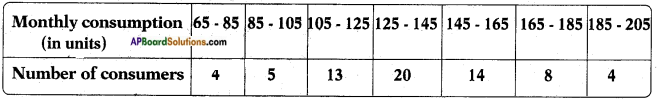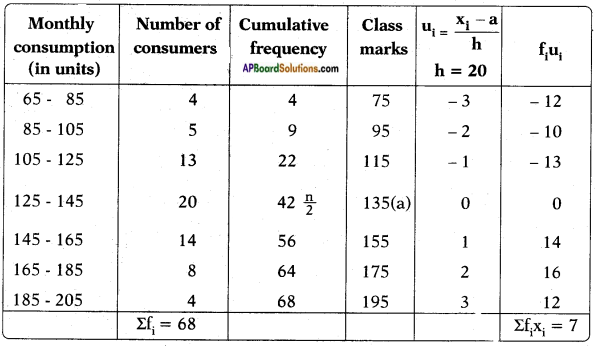Sum of the frequencies = 68
∴ $$\frac{n}{2}$$ = $$\frac{68}{2}$$ = 34
Hence median class = 125 – 145
Lower boundary of the median class, l = 125
cf – cumulative frequency of the class preceding the median class = 22
f – frequency of the median class = 20
h = class size = 20
Median = $$l+\frac{\left[\frac{\mathrm{n}}{2}-\mathrm{cf}\right]}{\mathrm{f}} \times \mathrm{h}$$
= 125 + $$\frac{[34-22]}{20}$$ × 20
∴ Median = 125 + 12 = 137
Maximum number of consumers lie in the class 125 – 145
Modal class is 125 -145
l – lower limit of the modal class =125
f1 – frequency of the modal class = 20
f0 – frequency of the class preceding the modal class =13
f2 – frequency of the class succeeding the modal class =14
h – size of the class = 20
Mode (Z) = $$l+\frac{f_{1}-f_{0}}{\left(f_{1}-f_{0}\right)+\left(f_{1}-f_{2}\right)} \times h$$
Mode (Z) = 125 + $$\frac{20-13}{(20-13)+(20-14)} \times 20$$
= 125 + $$\frac{7}{7+6}$$ × 20
= 125 + $$\frac{140}{13}$$
= 125 + 10.76923
∴ Mode = 135.769
Mean $$\overline{\mathrm{x}}=\mathrm{a}+\frac{\Sigma \mathrm{f}_{\mathrm{i}} \mathrm{u}_{\mathrm{i}}}{\Sigma \mathrm{f}_{\mathrm{i}}} \times \mathrm{h}$$
a = assumed mean = 135
∴ $$\overline{\mathbf{x}}$$ = 135 + $$\frac{7}{68}$$
= 135 + 0.102941
≃ 135.1
Mean, Median and Mode are approximately same in this case.Question 2.
If the median of 60 observations, given below is 28.5, find the values of x and y.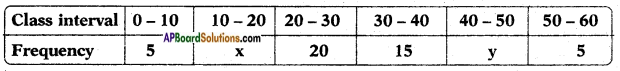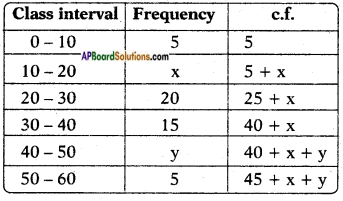Median = $$l+\frac{\left[\frac{\mathrm{n}}{2}-\mathrm{cf}\right]}{\mathrm{f}} \times \mathrm{h}$$
It is given that ∑f = n = 60
So, 45 + x + y = 60
x + y = 60 – 45 = 15
x + y = 15 ….. (1)
The median is 28.5 which lies be-tween 20 and 30.
Median class = 20 – 30
Lower boundary of the median class ‘l’ = 20
$$\frac{N}{2}$$ = $$\frac{60}{2}$$ = 30
cf – cumulative frequency = 5 + x
h = 10
Median = $$l+\frac{\left[\frac{\mathrm{n}}{2}-\mathrm{cf}\right]}{\mathrm{f}} \times \mathrm{h}$$
⇒ 28.5 = 20 + $$\frac{30-5-x}{20}$$ × 10
⇒ 28.5 = 20 + $$\frac{25-x}{2}$$
$$\frac{25-x}{2}$$ = 28.5 – 20 = 8.5
25 – x = 2 × 8.5
x = 25- 17 = 8
also from (1); x + y = 15
8 + y = 15
y = 7
∴ x = 8; y = 7.Question 3.
A life insurance agent found the following data about distribution of ages of 100 policy holders. Calculate the median age. [Policies are given only to persons having age 18 years onwards but less than 60 years.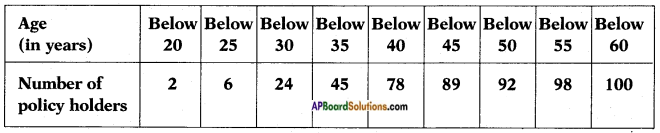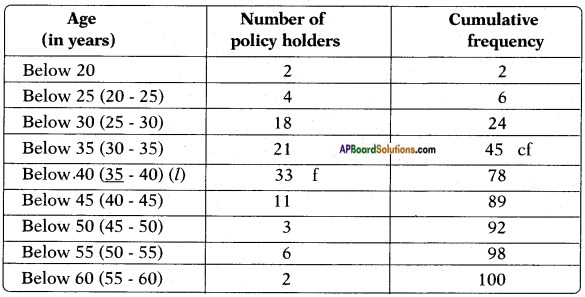The given distribution being of the less than type, 25, 30, 35, give the upper limits of corresponding class intervals. So the classes should be 20 – 25, 25 – 30, 30 – 35, ………. 55 – 60.
Observe that from the given distribution 2 persons with age less than 20.
i.e., frequency of the class below 20 is 2.
Now there are 6 persons with age less than 25 and 2 persons with age less than 20.
∴ The number of persons with age in the interval 20 – 25 is 6 – 2 = 4.
Similarly, the frequencies can be calculated as shown in table.
Number of observations = 100
n = 100
$$\frac{n}{2}$$ = $$\frac{100}{2}$$ = 50, which lies in the class 35-40
∴ 35 – 40 is the median class and lower boundary l = 35
cf = 45;
h = 5;
f = 33
Median = $$l+\frac{\left[\frac{\mathrm{n}}{2}-\mathrm{cf}\right]}{\mathrm{f}} \times \mathrm{h}$$
= 35 + $$\frac{50-45}{33}$$ × 5
= 35 + $$\frac{5}{33}$$ × 5
= 35 + 0.7575
= 35.7575
∴ Median ≃ 35.76Question 4.
The lengths of 40 leaves of a plant are measured correct to the nearest millimetre, and the data obtained is represented in the following table: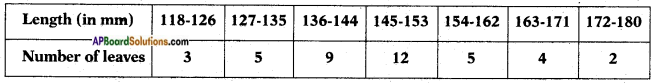Find the median length of the leaves. (Hint: The data needs to be converted to continuous classes for finding the median, since the formula assumes continuous classes. The classes then change to 117.5 – 126.5, 126.5 – 135.5,…, 171.5 – 180.5.)
Since the formula, Median = $$l+\frac{\left[\frac{\mathrm{n}}{2}-\mathrm{cf}\right]}{\mathrm{f}} \times \mathrm{h}$$ assumes continuous classes assumes continuous class, the data needs to be converted to continuous classes.
The classes then changes to 117.5 – 126.5; 126.5 – 133.5, …… 171.5 – 180.5.∑fi = n = 40
$$\frac{n}{2}$$ = $$\frac{40}{2}$$ = 20
$$\frac{n}{2}$$th observation lie in the class 144.5- 153.5
∴ Median class = 144.5 – 153.5
Lower boundary, l = 144.5
Frequency of the median class, f = 12
c.f. = 17
h = 9
∴ Median = $$l+\frac{\left[\frac{\mathrm{n}}{2}-\mathrm{cf}\right]}{\mathrm{f}} \times \mathrm{h}$$
= 144.5 + $$\frac{20-17}{12}$$ × 9
= 144.5 + $$\frac{3}{12}$$ × 9
= 144.5 + $$\frac{9}{4}$$
= 144.5 + 2.25
∴ Median length = 146.75 mm.Question 5.
The following table gives the distribution of the life-time of 400 neon lamps.Find the median life-time of a lamp.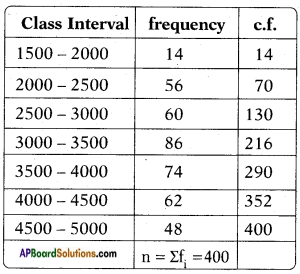Total observations are n = 400
$$\frac{n}{2}$$th observation i.e $$\frac{400}{2}$$ = 200
200 lies in the class 3000 – 3500
∴ Median class = 3000 – 3500
Lower boundary l = 3000
frequency of the median class f = 86
c.f = 130
Class size, h = 500
Median = $$l+\frac{\left[\frac{\mathrm{n}}{2}-\mathrm{cf}\right]}{\mathrm{f}} \times \mathrm{h}$$
= 3000 + $$\frac{200-130}{86}$$ × 500
= 3000 + $$\frac{70}{86}$$ × 500
= 3000 + 406.977
= 3406.98
∴ Median life ≃ 3406.98 hoursQuestion 6.
100 surnames were randomly picked up from a local telephone directory and the frequency distribution of the number of letters in the English alphabet in the surnames was obtained as follows.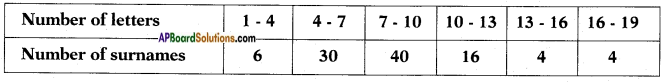Determine the median number of letters in the surnames. Find the mean number of letters in the surnames ? Also, find the modal size of the surnames.
Number of letters in the surnames.
Also find the modal size of the surnames.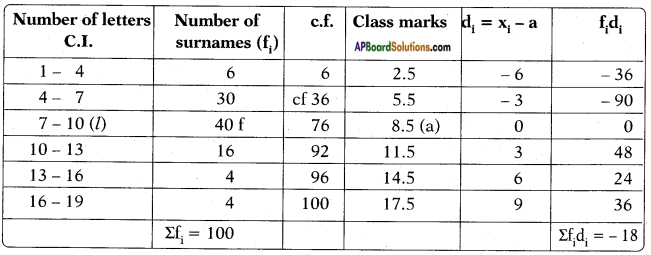Total observations are n = 100
$$\frac{n}{2}$$ = $$\frac{100}{2}$$ = 50
50 lies in the class 7 – 10
∴ Median class = 7 – 10
l – lower boundary = 7
f – frequency of the median class = 40
cf = 36
Class size h = 3
Median:
Median = $$l+\frac{\left[\frac{\mathrm{n}}{2}-\mathrm{cf}\right]}{\mathrm{f}} \times \mathrm{h}$$
= 7 + $$\frac{50-36}{40}$$ × 3
= 7 + $$\frac{14}{40}$$ × 3
= 7 + $$\frac{42}{40}$$
= 7 + 1.05
= 8.05
∴ Median = 8.05.

Mean:
Assumed mean, a = 8.5
Mean $$\overline{\mathrm{x}}=\mathrm{a}+\frac{\Sigma \mathrm{f}_{\mathrm{i}} \mathrm{d}_{\mathrm{i}}}{\Sigma \mathrm{f}_{\mathrm{i}}}$$
= 8.5 + $$\frac{(-18)}{100}$$
= 8.5 – 0.18
= 8.32
∴ Mean = 8.32.

Mode:
Maximum number of surnames = 40
∴ Modal class = 7-10
l – lower boundary of the modal class = 7
Mode (Z) = $$l+\frac{f_{1}-f_{0}}{\left(f_{1}-f_{0}\right)+\left(f_{1}-f_{2}\right)} \times h$$
l = 7; f1 = 40, f0 = 30, f2 = 16, h = 3
Mode (Z) = 7 + $$\frac{40-30}{(40-30)+(40-16)}$$ × 3
= 7 + $$\frac{10}{10+24}$$ × 3
= 7 + $$\frac{30}{34}$$
= 7 + 0.882
= 7.882

Median = 8.0.5; Mean = 8.32; Modal size = 7.88.Question 7.
The distribution below gives the weights of 30 students of a class. Find the median weight of the students.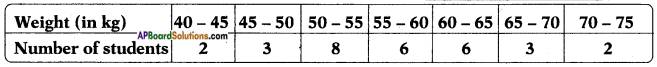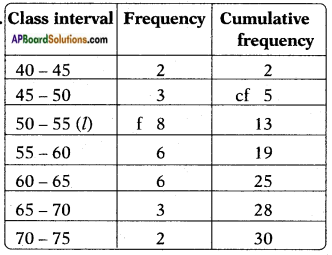Number of observations (n) = ∑fi
$$\frac{n}{2}$$ = $$\frac{30}{2}$$ = 15
15 lies in the class 50 – 55
∴ Median class = 50-55
l – lower boundary of the median class = 55
f – frequency of the median class = 8
c.f = 5
Class size h = 6
Median = $$l+\frac{\left[\frac{\mathrm{n}}{2}-\mathrm{cf}\right]}{\mathrm{f}} \times \mathrm{h}$$
= 50 + $$\frac{15-5}{8}$$ × 6
= 50 + 7.5
= 57.5
= 50 + 7.5 = 57.5
∴ Median weight = 57.5 kg.